You are currently offline. Some features of the site may not work correctly.

# Largest empty rectangle

Known as: Maximal empty rectangle, Maximum empty rectangle
In computational geometry, the largest empty rectangle problem, maximal empty rectangle problem or maximum empty rectangle problem, is the problem of… Expand
Wikipedia

## Papers overview

Semantic Scholar uses AI to extract papers important to this topic.
2013
2013
• GeoInformatica
• 2013
• Corpus ID: 44791462
Let S be a set of n points in a fixed axis-parallel rectangle \$R\subseteq \Re^{2}\$, i.e. in the two-dimensional space (2D… Expand
•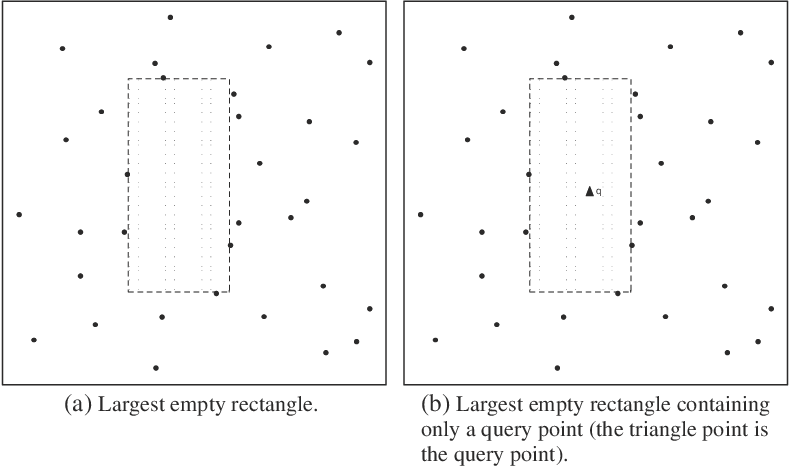•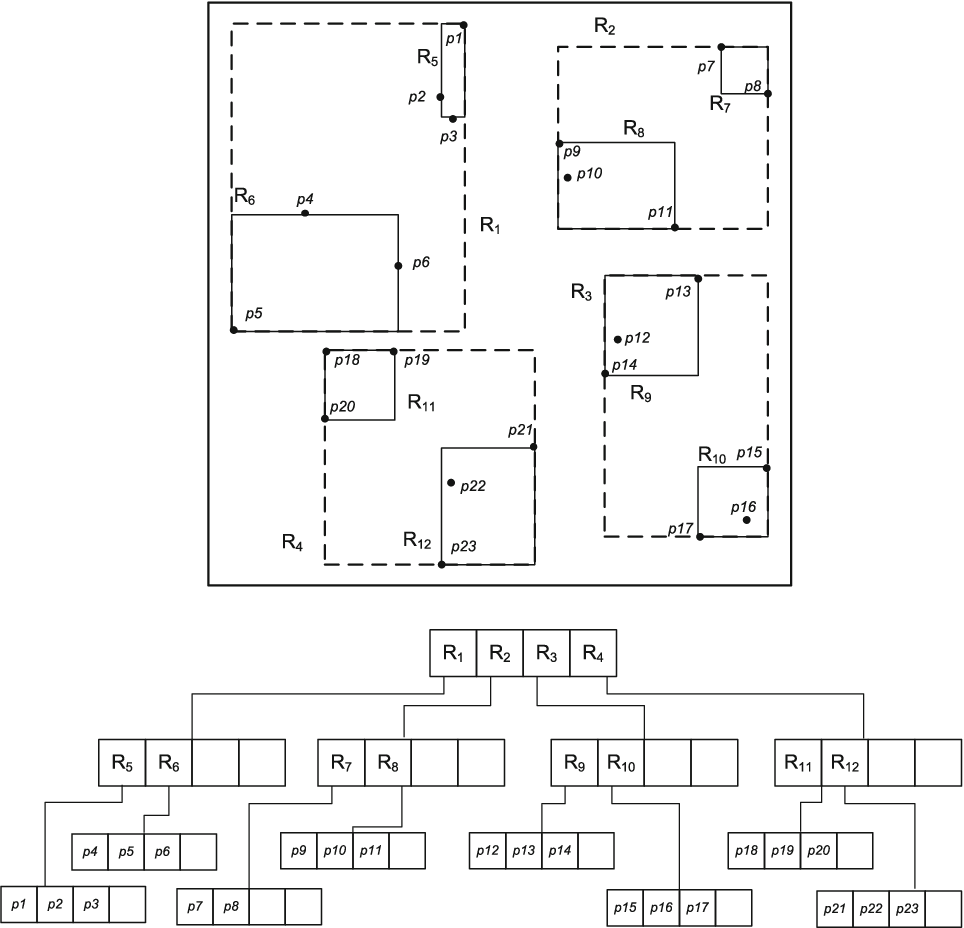•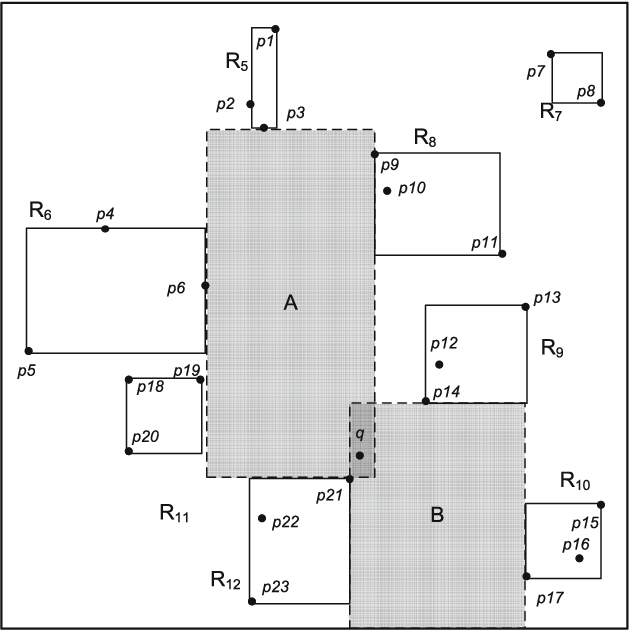•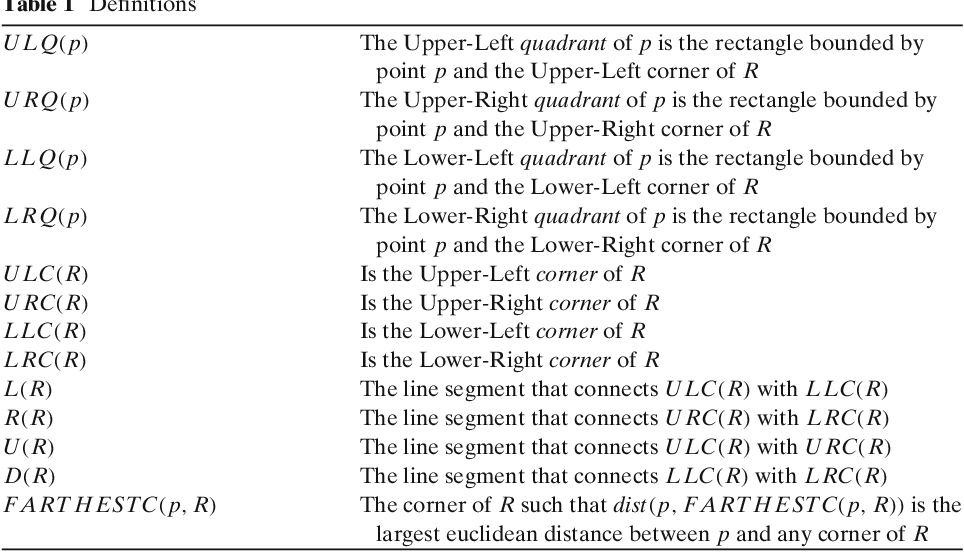•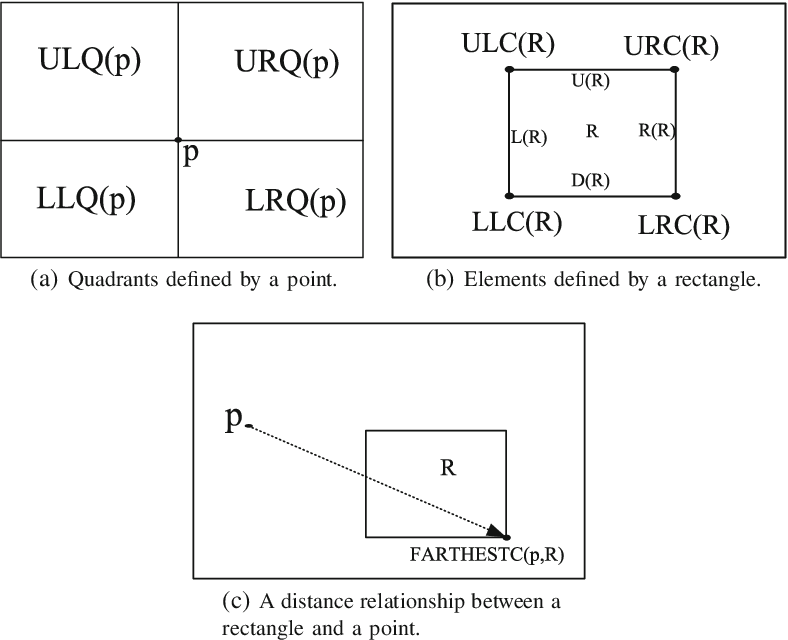Is this relevant?
2005
2005
A rectangleA and a setS ofn points inA are given. We present a new simple algorithm for the so-called largest empty rectangle… Expand
•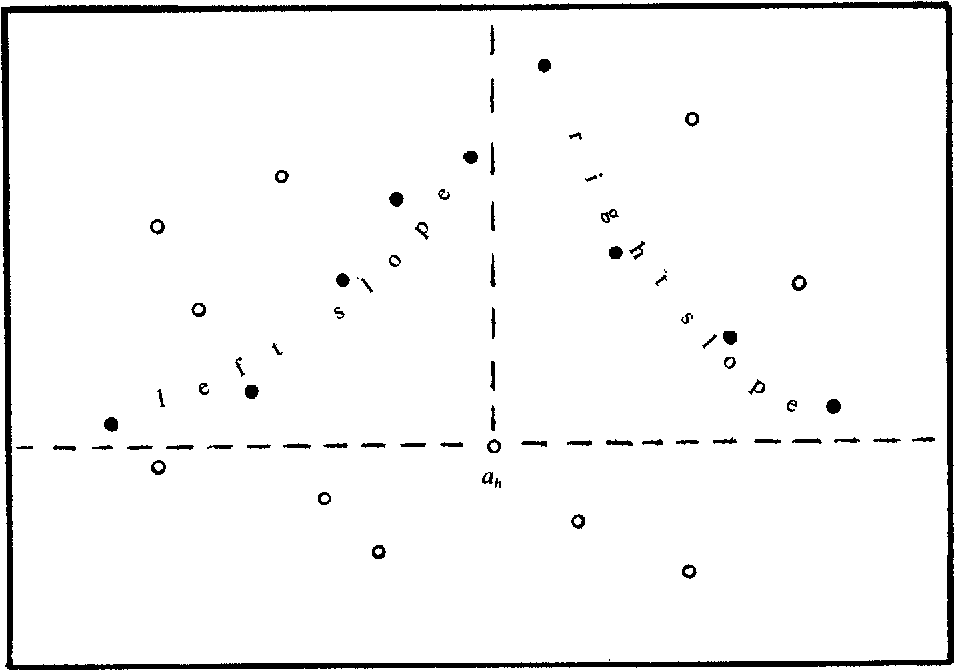•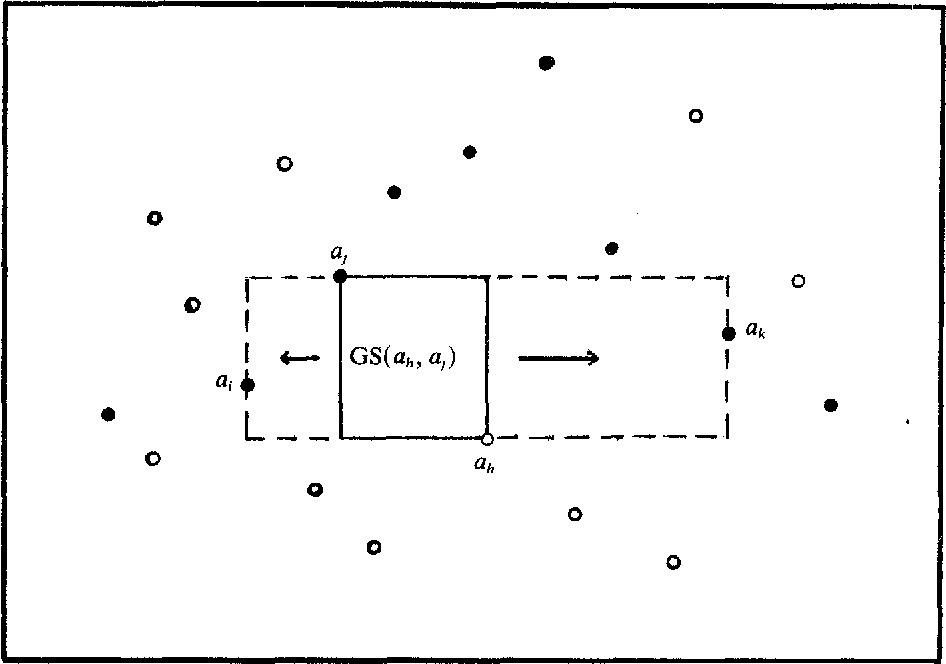•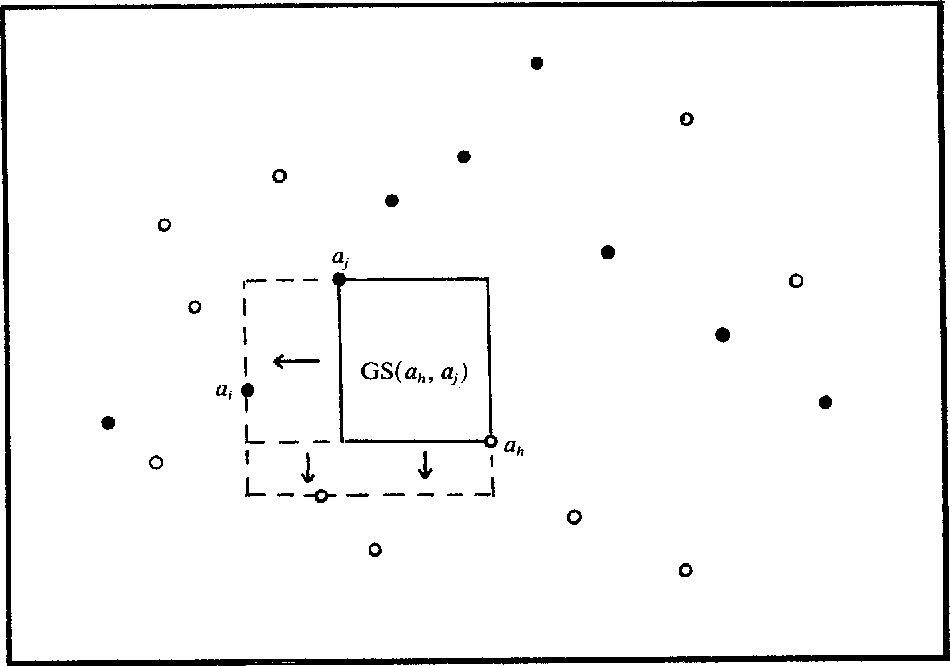Is this relevant?
2003
2003
• J. Algorithms
• 2003
• Corpus ID: 32029747
This work generalizes the classical problem of finding the largest empty rectangle among obstacles in 2D. Given a set P of n… Expand
Is this relevant?
1999
1999
• FSTTCS
• 1999
• Corpus ID: 31521684
This paper generalizes the classical MER problem in 2D. Given a set P of n points, here a maximal empty rectangle (MER) is… Expand
Is this relevant?
1994
1994
• FSTTCS
• 1994
• Corpus ID: 10866696
This paper outlines the following generalization of the classical maximal-empty-rectangle (MER) problem: given n arbitrarily… Expand
•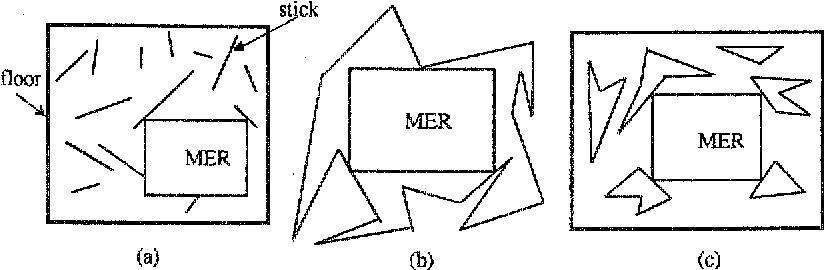•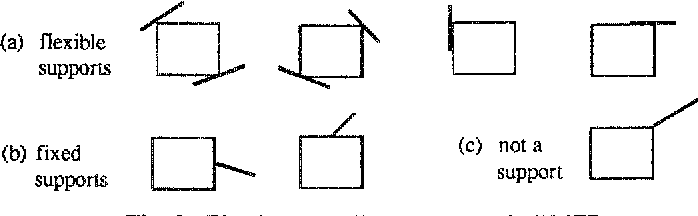•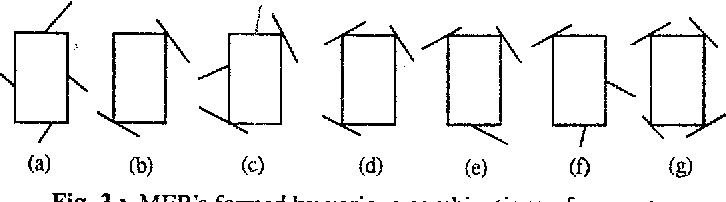•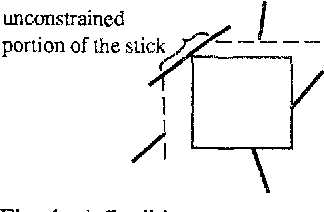•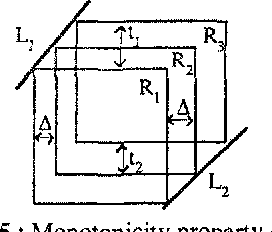Is this relevant?
1990
1990
• Frank Dehne
• J. Parallel Distributed Comput.
• 1990
• Corpus ID: 3139695
Abstract Given a rectangle R (with its edges parallel to the coordinate axes) containing a set S = { s1,…,sn} of n points in the… Expand
Is this relevant?
Highly Cited
1987
Highly Cited
1987
• SCG '87
• 1987
• Corpus ID: 18500442
We provide two algorithms for solving the following problem: Given a rectangle containing <italic>n</italic> points, compute the… Expand
•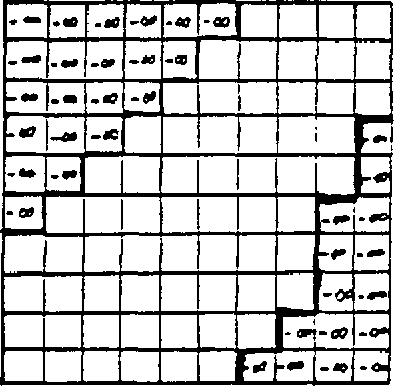•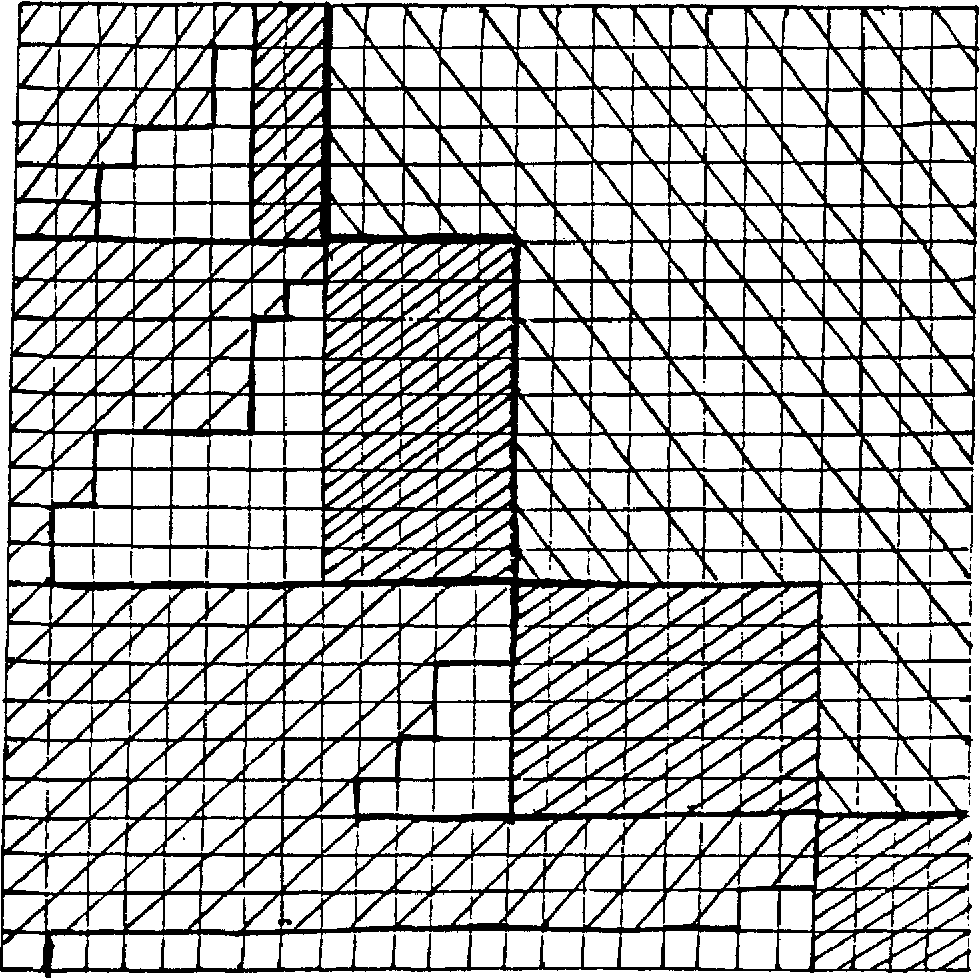Is this relevant?
1986
1986
• SIAM J. Comput.
• 1986
• Corpus ID: 37145219
We consider the following problem: Given a rectangle containing N points, find the largest area subrectangle with sides parallel… Expand
Is this relevant?
Highly Cited
1984
Highly Cited
1984
• STACS
• 1984
• Corpus ID: 3043444
We consider the following problem: Given a rectangle containing N points, find the largest area subrectangle with sides parallel… Expand
•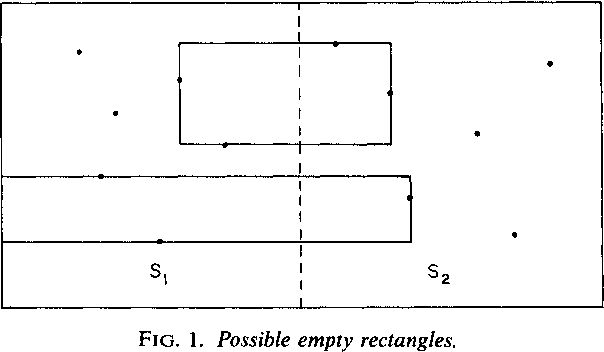•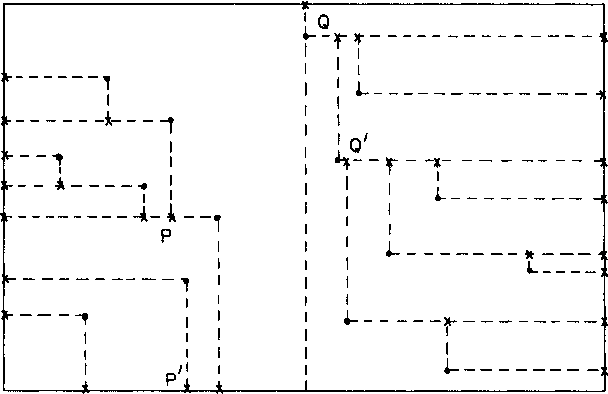•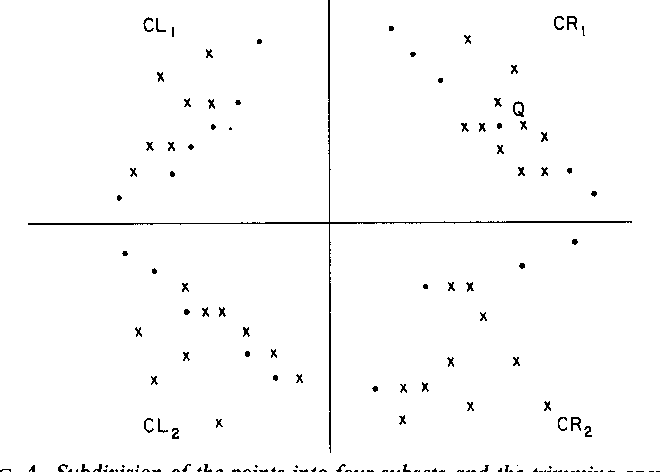•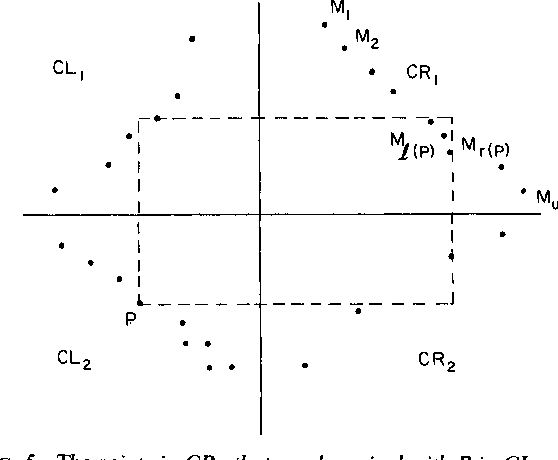•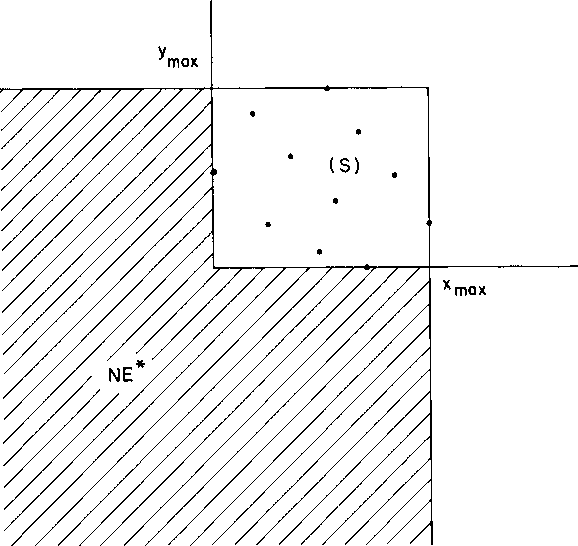Is this relevant?
Highly Cited
1984
Highly Cited
1984
• Discret. Appl. Math.
• 1984
• Corpus ID: 3180152
Abstract Given a rectangle A and a set S of n points in A, we consider the problem, called the maximum empty rectangle problem… Expand
•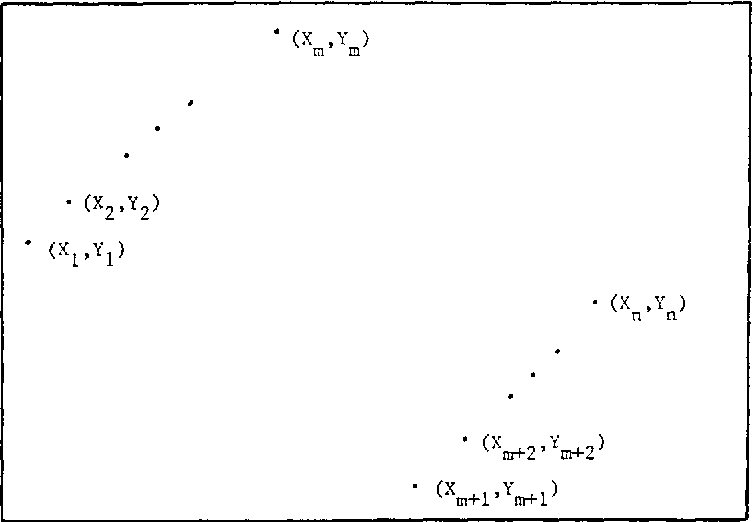•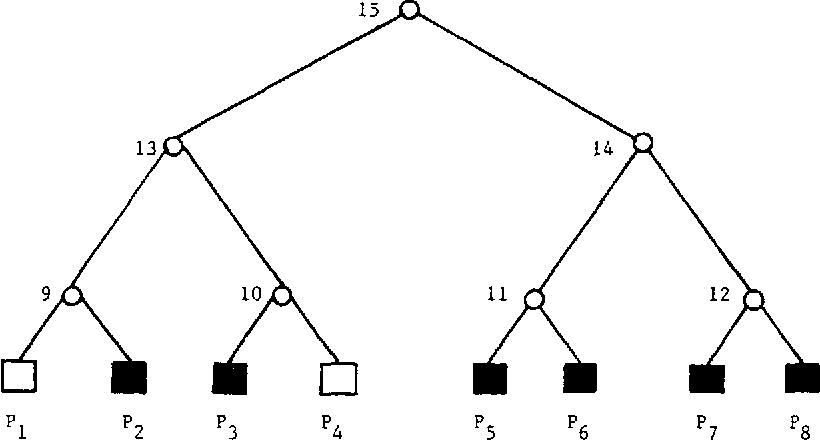•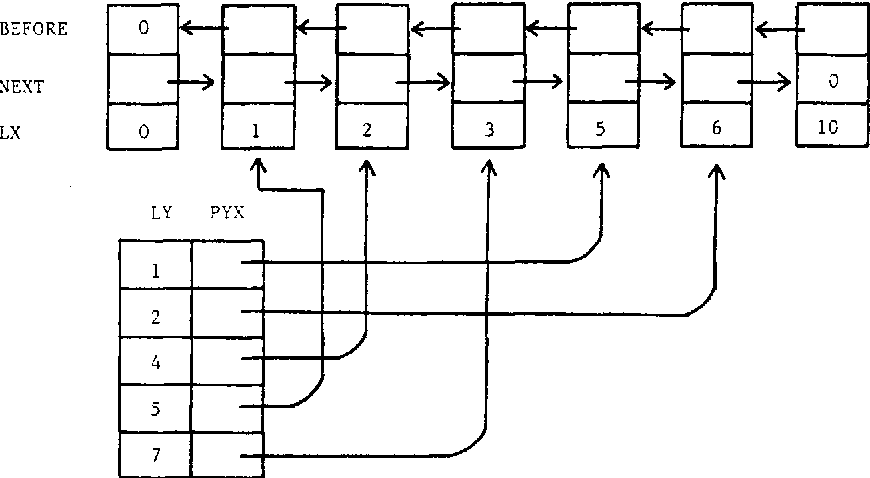Is this relevant?